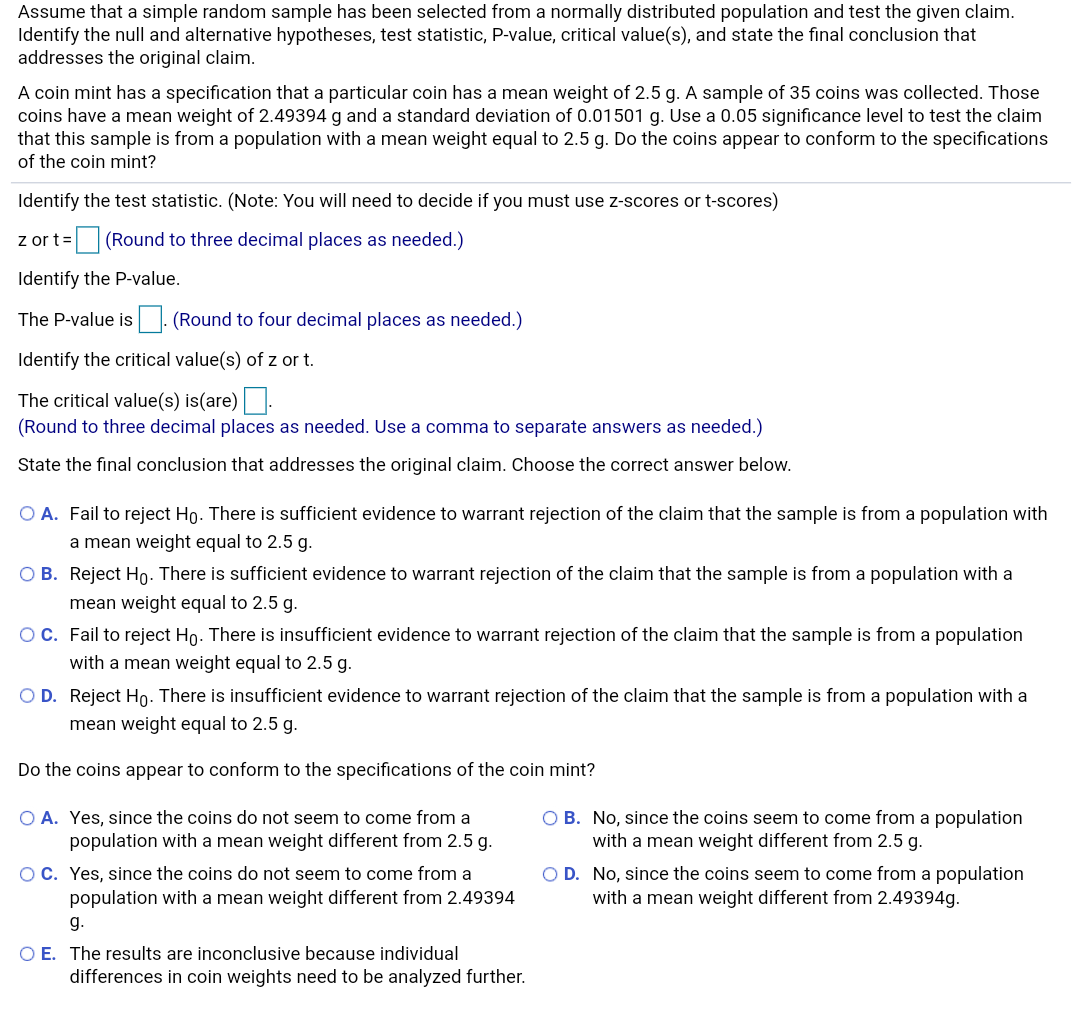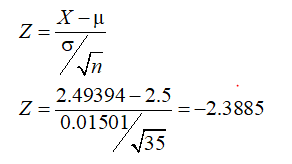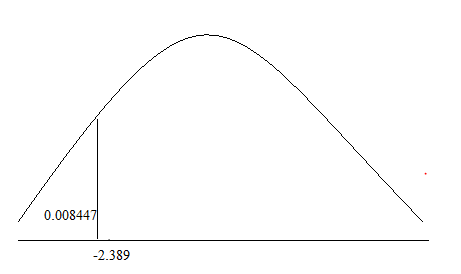# Assume that a simple random sample has been selected from a normally distributed population and test the given claim.Identify the null and alternative hypotheses, test statistic, P-value, critical value(s), and state the final conclusion thataddresses the original claim.A coin mint has a specification that a particular coin has a mean weight of 2.5 g. A sample of 35 coins was collected. Thosecoins have a mean weight of 2.49394 g and a standard deviation of 0.01501 g. Use a 0.05 significance level to test the claimthat this sample is from a population with a mean weight equal to 2.5 g. Do the coins appear to conform to the specificationsof the coin mint?Identify the test statistic. (Note: You will need to decide if you must use z-scores or t-scores)z or t=(Round to three decimal places as needed.)Identify the P-value.|. (Round to four decimal places as needed.)The P-value isIdentify the critical value(s) of z or t.The critical value(s) is(are) |.(Round to three decimal places as needed. Use a comma to separate answers as needed.)State the final conclusion that addresses the original claim. Choose the correct answer below.O A. Fail to reject Ho. There is sufficient evidence to warrant rejection of the claim that the sample is from a population witha mean weight equal to 2.5 g.O B. Reject Ho. There is sufficient evidence to warrant rejection of the claim that the sample is from a population with amean weight equal to 2.5 g.OC. Fail to reject Ho. There is insufficient evidence to warrant rejection of the claim that the sample is from a populationwith a mean weight equal to 2.5 g.O D. Reject Ho. There is insufficient evidence to warrant rejection of the claim that the sample is from a population with amean weight equal to 2.5 g.Do the coins appear to conform to the specifications of the coin mint?O A. Yes, since the coins do not seem to come from apopulation with a mean weight different from 2.5 g.O B. No, since the coins seem to come from a populationwith a mean weight different from 2.5 g.OC. Yes, since the coins do not seem to come from apopulation with a mean weight different from 2.49394O D. No, since the coins seem to come from a populationwith a mean weight different from 2.49394g.g.O E. The results are inconclusive because individualdifferences in coin weights need to be analyzed further.

Question
8 viewshelp_outlineImage TranscriptioncloseAssume that a simple random sample has been selected from a normally distributed population and test the given claim. Identify the null and alternative hypotheses, test statistic, P-value, critical value(s), and state the final conclusion that addresses the original claim. A coin mint has a specification that a particular coin has a mean weight of 2.5 g. A sample of 35 coins was collected. Those coins have a mean weight of 2.49394 g and a standard deviation of 0.01501 g. Use a 0.05 significance level to test the claim that this sample is from a population with a mean weight equal to 2.5 g. Do the coins appear to conform to the specifications of the coin mint? Identify the test statistic. (Note: You will need to decide if you must use z-scores or t-scores) z or t= (Round to three decimal places as needed.) Identify the P-value. |. (Round to four decimal places as needed.) The P-value is Identify the critical value(s) of z or t. The critical value(s) is(are) |. (Round to three decimal places as needed. Use a comma to separate answers as needed.) State the final conclusion that addresses the original claim. Choose the correct answer below. O A. Fail to reject Ho. There is sufficient evidence to warrant rejection of the claim that the sample is from a population with a mean weight equal to 2.5 g. O B. Reject Ho. There is sufficient evidence to warrant rejection of the claim that the sample is from a population with a mean weight equal to 2.5 g. OC. Fail to reject Ho. There is insufficient evidence to warrant rejection of the claim that the sample is from a population with a mean weight equal to 2.5 g. O D. Reject Ho. There is insufficient evidence to warrant rejection of the claim that the sample is from a population with a mean weight equal to 2.5 g. Do the coins appear to conform to the specifications of the coin mint? O A. Yes, since the coins do not seem to come from a population with a mean weight different from 2.5 g. O B. No, since the coins seem to come from a population with a mean weight different from 2.5 g. OC. Yes, since the coins do not seem to come from a population with a mean weight different from 2.49394 O D. No, since the coins seem to come from a population with a mean weight different from 2.49394g. g. O E. The results are inconclusive because individual differences in coin weights need to be analyzed further. fullscreen
check_circle

Step 1

Given
mean weight of coin = 2.5g
sample size = n = 35
Mean weight of coins in sample = 2.49394g
Standard deviation of coins in sample = 0.01501g
Level of significance = 0.05
Null Hypothesis : H0 : µ = 2.5g
Alternative Hypothesis : Ha : µ ≠ 2.5g

The sample size is 5 and given that population is normal, So we use a Z test and test statistic is calculated as shown belowTest statistic = -2.389 (rounded to three decimals)

Step 2

Using Excel and command NORM.DIST(x,mean,sd,cumulative) and plug in values we get
NORM.DIST(-2.389,0,1,1) = 0.008447
The probability to left of Z score -2.389 is 0.008447The hypothesis test is two tailed test. So the p value = 2*0.008447 = 0.016894 = 0.0169 (roun...

### Want to see the full answer?

See Solution

#### Want to see this answer and more?

Solutions are written by subject experts who are available 24/7. Questions are typically answered within 1 hour.*

See Solution
*Response times may vary by subject and question.
Tagged in

### Statistics